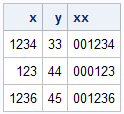This tutorial describes how we can add leading zeros to a numeric or character variable in SAS. It's one of the most frequently encountered data problem. It generally happens when we have some product codes that need to have leading zeros if the product code has a length less than defined length (let's say 10 characters). It sometimes become a daunting task when we merge two tables and the primary key is of different types and leading zeros is missing in one of the tables.

Create Sample Data

We would use the following dataset to demonstrate the way to add leading zeros to the numeric variable 'x'
data xy;
input x y;
cards;
1234 33
123 44
1236 45
;
run;
If the variable in which you want to add leading zeros contains only numeric values, we can simply use Zw.d format. In this case, we made length of the newly transformed variable as 6.
data temp;
set xy;
xx = put(x, z6.);
run;
z6. tells SAS to add 'k' number of leading zeros to the variable 'x' to maintain 6 as a length of the newly transformed variable 'xx'. In this case, 'k' = (6 - number of values in each observation in variable 'x'). In the first observation, it would add 2 leading zeros as the number of values is 4. However, it would add 3 leading zeros in second observation as it has 3 values.

The output is shown below :Output : Add leading zeros

Suppose you have a character variable in which you want to add leading zeros. In this case, we cannot use zw.d format as it only works for numeric variable.
data xy;
input x\$ y;
cards;
A1234 33
A123 44
A1236 45
;
run;
We need to keep 6 as length of the newly transformed variable.
data temp;
set xy;
xx = cats(repeat('0',6-length(x)-1), x);
proc print;
run;
CATS function is used to concatenate 0s with the variable 'x'. REPEAT function is used to repeat 0s. LENGTH function is used to determine the number of characters in the variable 'x'.  6 - length(x) -1 translates to ( 6- number of letters and values in the variable x - 1).OutputShare
Related PostsDeepanshu founded ListenData with a simple objective - Make analytics easy to understand and follow. He has over 10 years of experience in data science. During his tenure, he has worked with global clients in various domains like Banking, Insurance, Private Equity, Telecom and Human Resource.

1.Nice article, thank you man....keep up the good work..

1.2.Great stuff. Keep it up!

3.Thanks for that..!
It saves me lot of time..!
Great..

4.Hi, this was extremely helpful, but I don't think I still understand the -1 after length(x). Can you elaborate? Thank you!

1.REPEAT (argument,n) The REPEAT function returns a character value consisting of the first argument repeated n times. repeat('0',6-4-1) =repeat(0,1) means,0 will be repeated 1 time, hence 00 will be concatenated with X variable ( because length function determined length of x as 4 , so 2 times 0 will be concatenated )

5.This is excellent - straight forward and very easy to follow and implement. Thanks

6.Hi how are you. I have dataset,
id= 001,002,003,004,005
I want newid= 1,2,3,4,5
both are numeric. please resolve the issue

1.multily id with 1
eg : newid=id*1

it will remove all leading 0's if we have number values .

7.Thanks for the example. I have a similar problem but I only want to add the 0 if my string length is shorter than the wanted number of arguments. As in I have values "23A", "17", "265B" and I want them to be returned as "023A", "0017", and "265B". The above code seems to do the job. However I get a note saying that the values parsed in argument 2 are invalid. This is no surprice as I get negative values when I substract the extra 1. If I don't then I will get a 0 in front of everything. Can I avoid this 0?
I use the below code
cats(repeat('0',4-length(x)), x)

Next → ← Prev